# Equations Cast in Cylindrical Coordinates

 Spatial Operators in Cylindrical Coordinates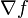$\nabla f$ =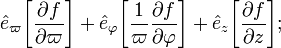${\hat{e}}_\varpi \biggl[ \frac{\partial f}{\partial\varpi} \biggr] + {\hat{e}}_\varphi {\biggl[ \frac{1}{\varpi} \frac{\partial f}{\partial\varphi} \biggr]} + {\hat{e}}_z \biggl[ \frac{\partial f}{\partial z} \biggr] ;$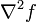$\nabla^2 f$ =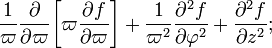$\frac{1}{\varpi} \frac{\partial }{\partial\varpi} \biggl[ \varpi \frac{\partial f}{\partial\varpi} \biggr] + {\frac{1}{\varpi^2} \frac{\partial^2 f}{\partial\varphi^2}} + \frac{\partial^2 f}{\partial z^2} ;$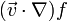$(\vec{v}\cdot\nabla)f$ =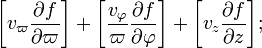$\biggl[ v_\varpi \frac{\partial f}{\partial\varpi} \biggr] + {\biggl[ \frac{v_\varphi}{\varpi} \frac{\partial f}{\partial\varphi} \biggr]} + \biggl[ v_z \frac{\partial f}{\partial z} \biggr] ;$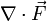$\nabla \cdot \vec{F}$ =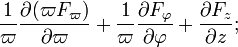$\frac{1}{\varpi} \frac{\partial (\varpi F_\varpi)}{\partial\varpi} + {\frac{1}{\varpi} \frac{\partial F_\varphi}{\partial\varphi}} + \frac{\partial F_z}{\partial z} ;$
 Vector Time-Derivatives in Cylindrical Coordinates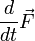$\frac{d}{dt}\vec{F}$ =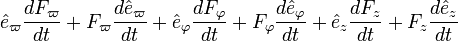${\hat{e}}_\varpi \frac{dF_\varpi}{dt} + F_\varpi \frac{d{\hat{e}}_\varpi}{dt} + {\hat{e}}_\varphi \frac{dF_\varphi}{dt} + F_\varphi \frac{d{\hat{e}}_\varphi}{dt} + {\hat{e}}_z \frac{dF_z}{dt} + F_z \frac{d{\hat{e}}_z}{dt}$ =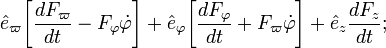${\hat{e}}_\varpi \biggl[ \frac{dF_\varpi}{dt} - F_\varphi \dot\varphi \biggr] + {\hat{e}}_\varphi \biggl[ \frac{dF_\varphi}{dt} + F_\varpi \dot\varphi \biggr] + {\hat{e}}_z \frac{dF_z}{dt} ;$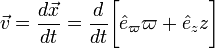$\vec{v} = \frac{d\vec{x}}{dt} = \frac{d}{dt}\biggl[ \hat{e}_\varpi \varpi + \hat{e}_z z \biggr]$ =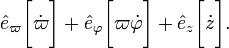${\hat{e}}_\varpi \biggl[ \dot\varpi \biggr] + {\hat{e}}_\varphi \biggl[ \varpi \dot\varphi \biggr] + {\hat{e}}_z \biggl[ \dot{z} \biggr] .$

## Governing Equations

Introducing the above expressions into the principal governing equations gives,

Equation of Continuity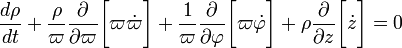$\frac{d\rho}{dt} + \frac{\rho}{\varpi} \frac{\partial}{\partial\varpi} \biggl[ \varpi \dot\varpi \biggr] + \frac{1}{\varpi} \frac{\partial}{\partial \varphi} \biggl[ \varpi \dot\varphi \biggr] + \rho \frac{\partial}{\partial z} \biggl[ \dot{z} \biggr] = 0$

Euler Equation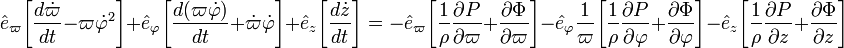${\hat{e}}_\varpi \biggl[ \frac{d \dot\varpi}{dt} - \varpi {\dot\varphi}^2 \biggr] + {\hat{e}}_\varphi \biggl[ \frac{d(\varpi\dot\varphi)}{dt} + \dot\varpi \dot\varphi \biggr] + {\hat{e}}_z \biggl[ \frac{d \dot{z}}{dt} \biggr] = - {\hat{e}}_\varpi \biggl[ \frac{1}{\rho}\frac{\partial P}{\partial\varpi} + \frac{\partial \Phi}{\partial\varpi}\biggr] - {\hat{e}}_\varphi \frac{1}{\varpi} \biggl[ \frac{1}{\rho}\frac{\partial P}{\partial \varphi} + \frac{\partial \Phi}{\partial \varphi} \biggr] - {\hat{e}}_z \biggl[ \frac{1}{\rho}\frac{\partial P}{\partial z} + \frac{\partial \Phi}{\partial z} \biggr]$

First Law of Thermodynamics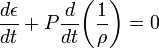$~\frac{d\epsilon}{dt} + P \frac{d}{dt} \biggl(\frac{1}{\rho}\biggr) = 0$

Poisson Equation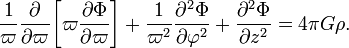$\frac{1}{\varpi} \frac{\partial }{\partial\varpi} \biggl[ \varpi \frac{\partial \Phi}{\partial\varpi} \biggr] + \frac{1}{\varpi^2} \frac{\partial^2 \Phi}{\partial \varphi^2} + \frac{\partial^2 \Phi}{\partial z^2} = 4\pi G \rho .$

## Eulerian Formulation

Each of the above simplified governing equations has been written in terms of Lagrangian time derivatives. An Eulerian formulation of each equation can be obtained by replacing each Lagrangian time derivative by its Eulerian counterpart. Specifically, for any scalar function, f,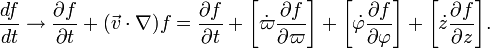$\frac{df}{dt} \rightarrow \frac{\partial f}{\partial t} + (\vec{v}\cdot \nabla)f = \frac{\partial f}{\partial t} + \biggl[ \dot\varpi \frac{\partial f}{\partial\varpi} \biggr] + \biggl[ \dot\varphi \frac{\partial f}{\partial\varphi} \biggr] + \biggl[ \dot{z} \frac{\partial f}{\partial z} \biggr] .$

Hence,

Equation of Continuity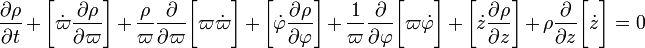$\frac{\partial\rho}{\partial t} + \biggl[ \dot\varpi \frac{\partial \rho}{\partial\varpi} \biggr] + \frac{\rho}{\varpi} \frac{\partial}{\partial\varpi} \biggl[ \varpi \dot\varpi \biggr] + \biggl[ \dot\varphi \frac{\partial \rho}{\partial\varphi} \biggr] + \frac{1}{\varpi} \frac{\partial}{\partial \varphi} \biggl[ \varpi \dot\varphi \biggr] + \biggl[ \dot{z} \frac{\partial \rho}{\partial z} \biggr] + \rho \frac{\partial}{\partial z} \biggl[ \dot{z} \biggr] = 0$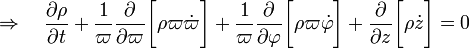$\Rightarrow ~~~ \frac{\partial\rho}{\partial t} + \frac{1}{\varpi} \frac{\partial}{\partial\varpi} \biggl[ \rho \varpi \dot\varpi \biggr] + \frac{1}{\varpi} \frac{\partial}{\partial \varphi} \biggl[ \rho \varpi \dot\varphi \biggr] + \frac{\partial}{\partial z} \biggl[ \rho \dot{z} \biggr] = 0$

Assuming that the initial (subscript i) configuration is axisymmetric and that, following perturbation, each physical parameter, Q, behaves according to the relation,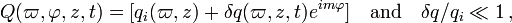$Q(\varpi, \varphi, z, t) = [q_i(\varpi, z) + \delta q(\varpi, z, t) e^{i m \varphi}] ~~~ \mathrm{and} ~~~ \delta q/q_i \ll 1 \, ,$

the linearized form of the continuity equation becomes:

 (This has been obtained by combining the expressions highlighted with a lightblue background color from the accompanying table.)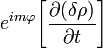$e^{im\varphi} \biggr[ \frac{\partial (\delta\rho) }{\partial t} \biggr]$ =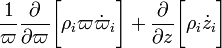$\frac{1}{\varpi} \frac{ \partial}{\partial\varpi} \biggl[ \rho_i \varpi \dot\varpi_i \biggr] + \frac{\partial}{\partial z} \biggl[ \rho_i \dot z_i \biggr]$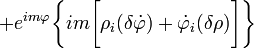$+ e^{im\varphi} \biggl\{ im \biggl[ \rho_i ( \delta\dot\varphi) + \dot\varphi_i (\delta\rho) \biggr] \biggr\}$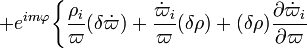$+ e^{im\varphi} \biggl\{ \frac{ \rho_i }{\varpi} ( \delta\dot\varpi ) + \frac{ \dot\varpi_i }{\varpi} ( \delta\rho ) + (\delta\rho) \frac{\partial {\dot\varpi_i} }{\partial\varpi}$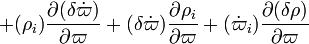$+ (\rho_i ) \frac{\partial ( \delta\dot\varpi)}{\partial\varpi} + ( \delta\dot\varpi) \frac{\partial \rho_i }{\partial\varpi} + ( {\dot\varpi_i} ) \frac{\partial (\delta\rho)}{\partial\varpi}$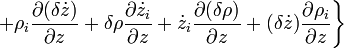$+ \rho_i \frac{\partial (\delta \dot z )}{\partial z} + \delta \rho \frac{\partial \dot z_i }{\partial z} + \dot z_i \frac{\partial (\delta \rho )}{\partial z} + (\delta \dot z )\frac{\partial \rho_i }{\partial z} \biggr\}$
 Linearize each term of the Continuity Equation assuming ...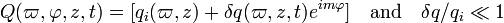$Q(\varpi, \varphi, z, t) = [q_i(\varpi, z) + \delta q(\varpi, z, t) e^{i m \varphi}] ~~~ \mathrm{and} ~~~ \delta q/q_i \ll 1$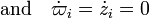$\mathrm{and} ~~~ \dot\varpi_i = \dot z_i = 0$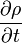$\frac{\partial\rho}{\partial t}$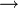$~~ \rightarrow ~~$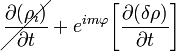$\cancel{ \frac{\partial (\rho_i) }{\partial t} } + e^{im\varphi} \biggr[ \frac{\partial (\delta\rho) }{\partial t} \biggr]$$~~ \rightarrow ~~$$e^{im\varphi} \biggr[ \frac{\partial (\delta\rho) }{\partial t} \biggr]$$~~~ \rightarrow ~~~$$e^{im\varphi} \biggr[ \frac{\partial (\delta\rho) }{\partial t} \biggr]$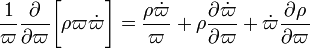$\frac{1}{\varpi} \frac{\partial}{\partial\varpi} \biggl[ \rho \varpi \dot\varpi \biggr] = \frac{\rho \dot\varpi}{\varpi} + \rho\frac{\partial \dot\varpi}{\partial\varpi} + \dot\varpi \frac{\partial \rho}{\partial\varpi}$$~~ \rightarrow ~~$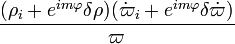$\frac{ (\rho_i + e^{im\varphi} \delta\rho) ( {\dot\varpi_i} + e^{im\varphi} \delta\dot\varpi)}{\varpi}$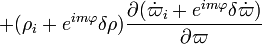$+ (\rho_i + e^{im\varphi} \delta\rho) \frac{\partial ( {\dot\varpi_i} + e^{im\varphi} \delta\dot\varpi)}{\partial\varpi}$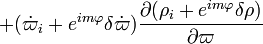$+ ( {\dot\varpi_i} + e^{im\varphi} \delta\dot\varpi) \frac{\partial (\rho_i + e^{im\varphi} \delta\rho)}{\partial\varpi}$$~~ \rightarrow ~~$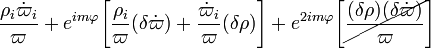$\frac{ \rho_i \dot\varpi_i}{\varpi} + e^{im\varphi} \biggl[ \frac{ \rho_i }{\varpi} ( \delta\dot\varpi ) + \frac{ \dot\varpi_i }{\varpi} ( \delta\rho ) \biggr] + e^{2im\varphi} \biggl[ \cancel{ \frac{ (\delta\rho) ( \delta\dot\varpi)}{\varpi} } \biggr]$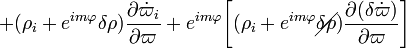$+ (\rho_i + e^{im\varphi} \delta\rho) \frac{\partial {\dot\varpi_i} }{\partial\varpi} + e^{im\varphi} \biggl[ (\rho_i + e^{im\varphi} \cancel{\delta\rho}) \frac{\partial ( \delta\dot\varpi)}{\partial\varpi} \biggr]$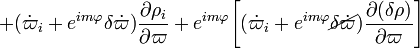$+ ( {\dot\varpi_i} + e^{im\varphi} \delta\dot\varpi) \frac{\partial \rho_i }{\partial\varpi} + e^{im\varphi}\biggl[ ( {\dot\varpi_i} + e^{im\varphi} \cancel{\delta\dot\varpi}) \frac{\partial (\delta\rho)}{\partial\varpi} \biggr]$$~~ \rightarrow ~~$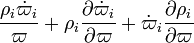$\frac{ \rho_i \dot\varpi_i}{\varpi} + \rho_i \frac{\partial \dot\varpi_i}{\partial \varpi} + \dot\varpi_i \frac{ \partial \rho_i}{\partial \varpi}$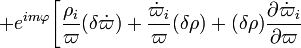$+ e^{im\varphi} \biggl[ \frac{ \rho_i }{\varpi} ( \delta\dot\varpi ) + \frac{ \dot\varpi_i }{\varpi} ( \delta\rho ) + (\delta\rho) \frac{\partial {\dot\varpi_i} }{\partial\varpi}$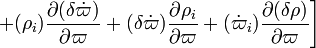$+ (\rho_i ) \frac{\partial ( \delta\dot\varpi)}{\partial\varpi} + ( \delta\dot\varpi) \frac{\partial \rho_i }{\partial\varpi} + ( {\dot\varpi_i} ) \frac{\partial (\delta\rho)}{\partial\varpi} \biggr]$$~~~~ \rightarrow ~~~~$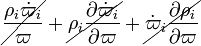$\cancel{ \frac{ \rho_i \dot\varpi_i}{\varpi} } + \cancel{ \rho_i \frac{\partial \dot\varpi_i}{\partial \varpi} } + \cancel{ \dot\varpi_i \frac{ \partial \rho_i}{\partial \varpi} }$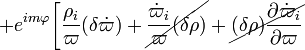$+ e^{im\varphi} \biggl[ \frac{ \rho_i }{\varpi} ( \delta\dot\varpi ) + \cancel{ \frac{ \dot\varpi_i }{\varpi} ( \delta\rho ) } + \cancel{ (\delta\rho) \frac{\partial {\dot\varpi_i} }{\partial\varpi} }$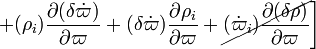$+ (\rho_i ) \frac{\partial ( \delta\dot\varpi)}{\partial\varpi} + ( \delta\dot\varpi) \frac{\partial \rho_i }{\partial\varpi} + \cancel{ ( {\dot\varpi_i} ) \frac{\partial (\delta\rho)}{\partial\varpi} } \biggr]$$~~~~ \rightarrow ~~~~$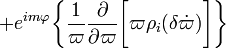$+ e^{im\varphi} \biggl\{ \frac{1}{\varpi} \frac{\partial}{\partial\varpi} \biggl[ \varpi \rho_i (\delta \dot\varpi) \biggr] \biggr\}$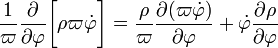$\frac{1}{\varpi} \frac{\partial}{\partial\varphi} \biggl[ \rho \varpi \dot\varphi \biggr] = \frac{\rho}{\varpi} \frac{\partial (\varpi \dot\varphi) }{\partial\varphi} + \dot\varphi \frac{\partial \rho}{\partial\varphi}$$~~ \rightarrow ~~$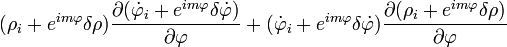$(\rho_i + e^{im\varphi} \delta\rho) \frac{\partial ( {\dot\varphi_i} + e^{im\varphi} \delta\dot\varphi)}{\partial\varphi} + ( {\dot\varphi_i} +e^{im\varphi} \delta\dot\varphi) \frac{\partial (\rho_i + e^{im\varphi} \delta\rho)}{\partial\varphi}$$~~ \rightarrow ~~$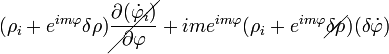$(\rho_i + e^{im\varphi} \delta\rho) \cancel{ \frac{\partial ( {\dot\varphi_i} )}{\partial\varphi} } + im e^{im\varphi} (\rho_i + e^{im\varphi} \cancel{ \delta\rho })( \delta\dot\varphi)$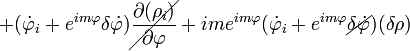$+ ( {\dot\varphi_i} +e^{im\varphi} \delta\dot\varphi) \cancel{ \frac{\partial (\rho_i )}{\partial\varphi} } + im e^{im\varphi} ( {\dot\varphi_i} +e^{im\varphi} \cancel{ \delta\dot\varphi }) (\delta\rho)$$~~ \rightarrow ~~$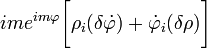$im e^{im\varphi} \biggl[ \rho_i ( \delta\dot\varphi) + \dot\varphi_i (\delta\rho) \biggr]$$~~~ \rightarrow ~~~$$im e^{im\varphi} \biggl[ \rho_i ( \delta\dot\varphi) + \dot\varphi_i (\delta\rho) \biggr]$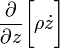$\frac{\partial}{\partial z} \biggl[ \rho \dot{z} \biggr]$$~~ \rightarrow ~~$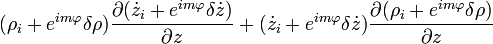$(\rho_i + e^{im\varphi} \delta\rho) \frac{\partial ( {\dot{z}_i} + e^{im\varphi} \delta\dot{z})}{\partial z} + ( {\dot{z}_i} +e^{im\varphi} \delta\dot{z}) \frac{\partial (\rho_i + e^{im\varphi} \delta\rho)}{\partial z}$$~~ \rightarrow ~~$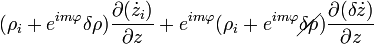$(\rho_i + e^{im\varphi} \delta\rho) { \frac{\partial ( {\dot{z}_i} )}{\partial z} } + e^{im\varphi} (\rho_i + e^{im\varphi} \cancel{{ \delta\rho } } ) \frac{\partial ( \delta\dot{z})}{\partial z}$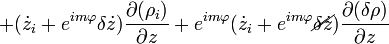$+ ( {\dot{z}_i} +e^{im\varphi} \delta\dot{z}) \frac{\partial (\rho_i )}{\partial z} + e^{im\varphi} ( {\dot{z}_i} +e^{im\varphi} \cancel{ \delta\dot{z} } ) \frac{\partial (\delta\rho)}{\partial z}$$~~ \rightarrow ~~$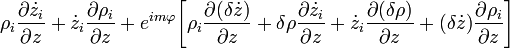$\rho_i \frac{\partial \dot z_i }{\partial z} + \dot{z}_i \frac{\partial \rho_i}{\partial z} + e^{im\varphi} \biggl[ \rho_i \frac{\partial (\delta \dot z )}{\partial z} + \delta \rho \frac{\partial \dot z_i }{\partial z} + \dot z_i \frac{\partial (\delta \rho )}{\partial z} + (\delta \dot z )\frac{\partial \rho_i }{\partial z} \biggr]$$~~~ \rightarrow ~~~$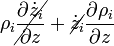$\rho_i \cancel{ \frac{\partial \dot z_i }{\partial z} } + \cancel{ \dot{z}_i } \frac{\partial \rho_i}{\partial z}$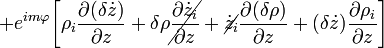$+ e^{im\varphi} \biggl[ \rho_i \frac{\partial (\delta \dot z )}{\partial z} + \delta \rho \cancel{ \frac{\partial \dot z_i }{\partial z} } + \cancel{ \dot z_i } \frac{\partial (\delta \rho )}{\partial z} + (\delta \dot z )\frac{\partial \rho_i }{\partial z} \biggr]$$~~~ \rightarrow ~~~$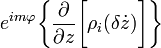$e^{im\varphi} \biggl\{ \frac{\partial}{\partial z} \biggl[ \rho_i (\delta \dot z ) \biggr] \biggr\}$ Combining all terms:$~~~ \rightarrow ~~~$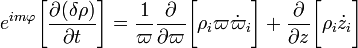$e^{im\varphi} \biggr[ \frac{\partial (\delta\rho) }{\partial t} \biggr] = \frac{1}{\varpi} \frac{ \partial}{\partial\varpi} \biggl[ \rho_i \varpi \dot\varpi_i \biggr] + \frac{\partial}{\partial z} \biggl[ \rho_i \dot z_i \biggr]$$+ e^{im\varphi} \biggl\{ \frac{ \rho_i }{\varpi} ( \delta\dot\varpi ) + \frac{ \dot\varpi_i }{\varpi} ( \delta\rho ) + (\delta\rho) \frac{\partial {\dot\varpi_i} }{\partial\varpi}$$+ (\rho_i ) \frac{\partial ( \delta\dot\varpi)}{\partial\varpi} + ( \delta\dot\varpi) \frac{\partial \rho_i }{\partial\varpi} + ( {\dot\varpi_i} ) \frac{\partial (\delta\rho)}{\partial\varpi}$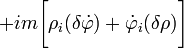$+ im \biggl[ \rho_i ( \delta\dot\varphi) + \dot\varphi_i (\delta\rho) \biggr]$$+ \rho_i \frac{\partial (\delta \dot z )}{\partial z} + \delta \rho \frac{\partial \dot z_i }{\partial z} + \dot z_i \frac{\partial (\delta \rho )}{\partial z} + (\delta \dot z )\frac{\partial \rho_i }{\partial z} \biggr\}$$~~~ \rightarrow ~~~$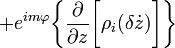$+ e^{im\varphi} \biggl\{ \frac{\partial}{\partial z} \biggl[ \rho_i (\delta \dot z ) \biggr] \biggr\}$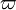$\varpi$ Component of Euler Equation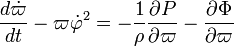$\frac{d \dot\varpi}{dt} - \varpi {\dot\varphi}^2 = - \frac{1}{\rho}\frac{\partial P}{\partial\varpi} - \frac{\partial \Phi}{\partial\varpi}$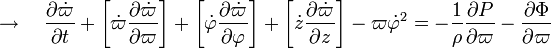$\rightarrow ~~~ \frac{\partial \dot\varpi}{\partial t} + \biggl[ \dot\varpi \frac{\partial \dot\varpi}{\partial\varpi} \biggr] + \biggl[ \dot\varphi \frac{\partial \dot\varpi}{\partial\varphi} \biggr] + \biggl[ \dot{z} \frac{\partial \dot\varpi}{\partial z} \biggr] - \varpi {\dot\varphi}^2 = - \frac{1}{\rho}\frac{\partial P}{\partial\varpi} - \frac{\partial \Phi}{\partial\varpi}$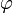$\varphi$ Component of Euler Equation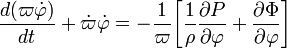$\frac{d (\varpi\dot\varphi) }{dt} + \dot\varpi \dot\varphi = - \frac{1}{\varpi} \biggl[ \frac{1}{\rho}\frac{\partial P}{\partial \varphi} + \frac{\partial \Phi}{\partial \varphi} \biggr]$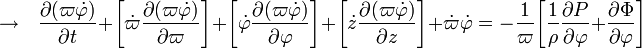$\rightarrow ~~~ \frac{\partial (\varpi\dot\varphi)}{\partial t} + \biggl[ \dot\varpi \frac{\partial (\varpi\dot\varphi)}{\partial\varpi} \biggr] + \biggl[ \dot\varphi \frac{\partial (\varpi\dot\varphi)}{\partial\varphi} \biggr] + \biggl[ \dot{z} \frac{\partial (\varpi\dot\varphi)}{\partial z} \biggr] + \dot\varpi \dot\varphi = - \frac{1}{\varpi} \biggl[ \frac{1}{\rho}\frac{\partial P}{\partial \varphi} + \frac{\partial \Phi}{\partial \varphi} \biggr]$

z Component of Euler Equation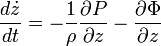$\frac{d \dot{z} }{dt} = - \frac{1}{\rho}\frac{\partial P}{\partial z} - \frac{\partial \Phi}{\partial z}$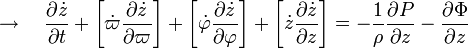$\rightarrow ~~~ \frac{\partial \dot{z}}{\partial t} + \biggl[ \dot\varpi \frac{\partial \dot{z}}{\partial\varpi} \biggr] + \biggl[ \dot\varphi \frac{\partial \dot{z}}{\partial\varphi} \biggr] +\biggl[ \dot{z} \frac{\partial \dot{z}}{\partial z} \biggr] = - \frac{1}{\rho}\frac{\partial P}{\partial z} - \frac{\partial \Phi}{\partial z}$